Next: Vector velocity and vector Up: Motion in 3 dimensions Previous: Scalar multiplication

## Diagonals of a parallelogram

The use of vectors is very well illustrated by the following rather famous proof that the diagonals of a parallelogram mutually bisect one another.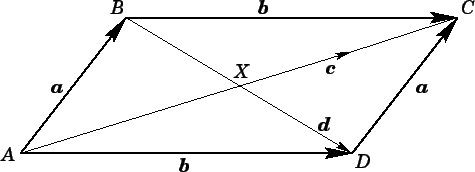Suppose that the quadrilateral ABCD in Fig. 13 is a parallelogram. It follows that the opposite sides of ABCD can be represented by the same vectors,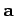and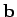: this merely indicates that these sides are of equal length and are parallel (i.e., they point in the same direction). Note that Fig. 13 illustrates an important point regarding vectors. Although vectors possess both a magnitude (length) and a direction, they possess no intrinsic position information. Thus, since sides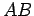and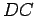are parallel and of equal length, they can be represented by the same vector, despite the fact that they are in different places on the diagram.

The diagonal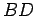in Fig. 13 can be represented vectorially as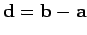. Likewise, the diagonal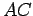can be written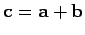. The displacement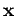(say) of the centroid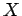from pointcan be written in one of two different ways: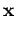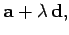(38)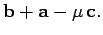(39)

Equation (38) is interpreted as follows: in order to get from pointto point, first move to point(along vector), then move along diagonal(along vector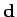) for an unknown fraction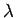of its length. Equation (39) is interpreted as follows: in order to get from pointto point, first move to point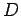(along vector), then move to point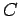(along vector), finally move along diagonal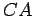(along vector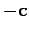) for an unknown fraction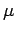of its length. Sincerepresents the same point in Eqs. (38) and (39), we can equate these two expressions to give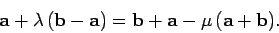(40)

Now vectorsandpoint in different directions, so the only way in which the above expression can be satisfied, in general, is if the coefficients ofandmatch on either side of the equality sign. Thus, equating coefficients ofand, we obtain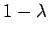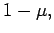(41)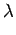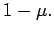(42)

It follows that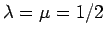. In other words, the centroidis located at the halfway points of diagonalsand: i.e., the diagonals mutually bisect one another.Next: Vector velocity and vector Up: Motion in 3 dimensions Previous: Scalar multiplication
Richard Fitzpatrick 2006-02-02